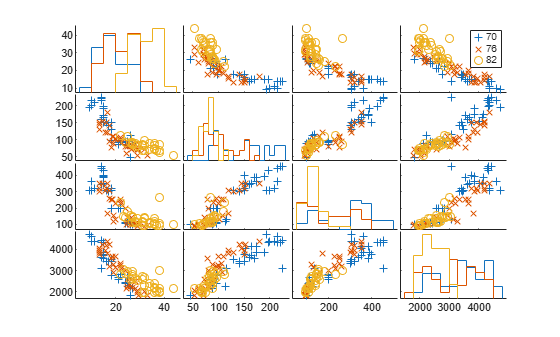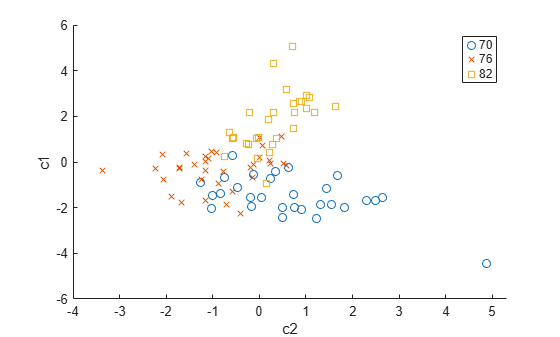## MANOVA

### Introduction to MANOVA

The analysis of variance technique in Perform One-Way ANOVA takes a set of grouped data and determine whether the mean of a variable differs significantly among groups. Often there are multiple response variables, and you are interested in determining whether the entire set of means is different from one group to the next. There is a multivariate version of analysis of variance that can address the problem.

### ANOVA with Multiple Responses

The `carsmall` data set has measurements on a variety of car models from the years 1970, 1976, and 1982. Suppose you are interested in whether the characteristics of the cars have changed over time.

```load carsmall whos```
``` Name Size Bytes Class Attributes Acceleration 100x1 800 double Cylinders 100x1 800 double Displacement 100x1 800 double Horsepower 100x1 800 double MPG 100x1 800 double Mfg 100x13 2600 char Model 100x33 6600 char Model_Year 100x1 800 double Origin 100x7 1400 char Weight 100x1 800 double ```

Four of these variables (`Acceleration`, `Displacement`, `Horsepower`, and `MPG`) are continuous measurements on individual car models. The variable `Model_Year` indicates the year in which the car was made. You can create a grouped plot matrix of these variables using the `gplotmatrix` function.

Create a grouped plot matrix of these variables using the `gplotmatrix` function.

```x = [MPG Horsepower Displacement Weight]; gplotmatrix(x,[],Model_Year,[],'+xo')```(When the second argument of `gplotmatrix` is empty, the function graphs the columns of the `x` argument against each other, and places histograms along the diagonals. The empty fourth argument produces a graph with the default colors. The fifth argument controls the symbols used to distinguish between groups.)

It appears the cars do differ from year to year. The upper right plot, for example, is a graph of `MPG` versus `Weight`. The 1982 cars appear to have higher mileage than the older cars, and they appear to weigh less on average. But as a group, are the three years significantly different from one another? The `manova1` function can answer that question.

`[d,p,stats] = manova1(x,Model_Year)`
```d = 2 ```
```p = 2×1 10-6 × 0.0000 0.1141 ```
```stats = struct with fields: W: [4x4 double] B: [4x4 double] T: [4x4 double] dfW: 90 dfB: 2 dfT: 92 lambda: [2x1 double] chisq: [2x1 double] chisqdf: [2x1 double] eigenval: [4x1 double] eigenvec: [4x4 double] canon: [100x4 double] mdist: [2.8187 0.7899 0.6187 0.4050 0.8538 4.2337 6.0117 4.9600 ... ] gmdist: [3x3 double] gnames: {3x1 cell} ```

The `manova1` function produces three outputs:

• The first output `d` is an estimate of the dimension of the group means. If the means were all the same, the dimension would be 0, indicating that the means are at the same point. If the means differed but fell along a line, the dimension would be 1. In the example the dimension is 2, indicating that the group means fall in a plane but not along a line. This is the largest possible dimension for the means of three groups.

• The second output `p` is a vector of p-values for a sequence of tests. The first p-value tests whether the dimension is 0, the next whether the dimension is 1, and so on. In this case both p-values are small. That's why the estimated dimension is 2.

• The third output `stats` is a structure containing several fields, described in the following section.

Fields of the `stats` Structure

The `W`, `B`, and `T` fields are matrix analogs to the within, between, and total sums of squares in ordinary one-way analysis of variance. The next three fields are the degrees of freedom for these matrices. Fields `lambda`, `chisq`, and `chisqdf` are the ingredients of the test for the dimensionality of the group means. (The p-values for these tests are the first output argument of `manova1`.)

The next three fields are used to do a canonical analysis. Recall that in Principal Component Analysis (PCA) you look for the combination of the original variables that has the largest possible variation. In multivariate analysis of variance, you instead look for the linear combination of the original variables that has the largest separation between groups. It is the single variable that would give the most significant result in a univariate one-way analysis of variance. Having found that combination, you next look for the combination with the second highest separation, and so on.

The `eigenvec` field is a matrix that defines the coefficients of the linear combinations of the original variables. The `eigenval` field is a vector measuring the ratio of the between-group variance to the within-group variance for the corresponding linear combination. The `canon` field is a matrix of the canonical variable values. Each column is a linear combination of the mean-centered original variables, using coefficients from the `eigenvec` matrix.

```c1 = stats.canon(:,1); c2 = stats.canon(:,2);```

Plot the grouped scatter plot of the first two canonical variables.

```figure gscatter(c2,c1,Model_Year,[],'oxs')```A grouped scatter plot of the first two canonical variables shows more separation between groups then a grouped scatter plot of any pair of original variables. In this example, it shows three clouds of points, overlapping but with distinct centers. One point in the bottom right sits apart from the others. You can mark this point on the plot using the `gname` function.

Roughly speaking, the first canonical variable, `c1`, separates the 1982 cars (which have high values of `c1`) from the older cars. The second canonical variable, `c2`, reveals some separation between the 1970 and 1976 cars.

The final two fields of the `stats` structure are Mahalanobis distances. The `mdist` field measures the distance from each point to its group mean. Points with large values may be outliers. In this data set, the largest outlier is the one in the scatter plot, the Buick Estate station wagon. (Note that you could have supplied the model name to the `gname` function above if you wanted to label the point with its model name rather than its row number.)

Find the largest distance from the group mean.

`max(stats.mdist)`
```ans = 31.5273 ```

Find the point that has the largest distance from the group mean.

`find(stats.mdist == ans)`
```ans = 20 ```

Find the car model that corresponds to the largest distance from the group mean.

`Model(20,:)`
```ans = 'buick estate wagon (sw) ' ```

The `gmdist` field measures the distances between each pair of group means. Examine the group means using `grpstats`.

`grpstats(x, Model_Year)`
```ans = 3×4 103 × 0.0177 0.1489 0.2869 3.4413 0.0216 0.1011 0.1978 3.0787 0.0317 0.0815 0.1289 2.4535 ```

Find the distances between the each pair of group means.

`stats.gmdist`
```ans = 3×3 0 3.8277 11.1106 3.8277 0 6.1374 11.1106 6.1374 0 ```

As might be expected, the multivariate distance between the extreme years 1970 and 1982 (11.1) is larger than the difference between more closely spaced years (3.8 and 6.1). This is consistent with the scatter plots, where the points seem to follow a progression as the year changes from 1970 through 1976 to 1982. If you had more groups, you might find it instructive to use the `manovacluster` function to draw a diagram that presents clusters of the groups, formed using the distances between their means.